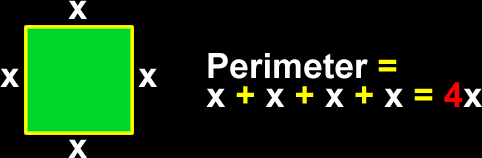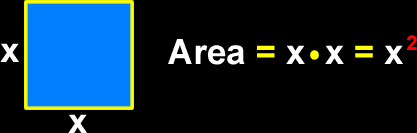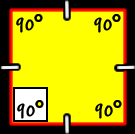# The Properties of a Square

Definitions and formulas for the perimeter of a square, the area of a square,  how to find the length of the diagonal of a square, properties of the diagonals of a square
Just scroll down or click on what you want and I'll scroll down for you!

 perimeter of a square area of a square sides and anglesof a square diagonal of a square central angle of a square facts about squares

 The perimeter of a square: To find the perimeter of a square, just add up all the lengths of the sides:The area of a square: To find the area of a square, multiply the lengths of two sides together...  Another way to say this is to say "square the length of a side!"  Get it?  Square!The sides and angles of a square:The sides of a square are all congruent (the same length.) The angles of a square are all congruent (the same size and measure.) Remember that a 90 degree angle is  called a "right angle."  So, a square has four right angles. Opposite angles of a square are congruent.Opposite sides of a square are congruent.Opposite sides of a square are parallel.